# Test: Regular Expressions & Languages- 2

## 30 Questions MCQ Test GATE Computer Science Engineering(CSE) 2023 Mock Test Series | Test: Regular Expressions & Languages- 2

Description
Attempt Test: Regular Expressions & Languages- 2 | 30 questions in 90 minutes | Mock test for Computer Science Engineering (CSE) preparation | Free important questions MCQ to study GATE Computer Science Engineering(CSE) 2023 Mock Test Series for Computer Science Engineering (CSE) Exam | Download free PDF with solutions
QUESTION: 1

### Consider the regular grammar below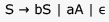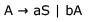The Myhill-Nerode equivalence classes for the language generated by the grammar are

Solution:

The given grammar generates all string over the alphabet {a,b}  which have an even number of 's.
The given right-linear grammar can be converted to the following DFA.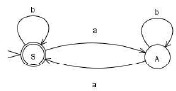QUESTION: 2

### Consider the alphabet ∑ = {0,1} , the null/empty string λ  and the set of strings X0,X1 and X2  generated by the corresponding non-terminals of a regular grammar. X0,X1 and X2  are related as follows.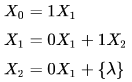Which one of the following choices precisely represents the strings in X0?

Solution:

Here we have little different version of Arden's Theorem

if we have R= PR + Q   then it has a solution  R = P*Q

Proof : R= PR+ Q
= P(PR+Q)+Q    (by putting R= PR+Q)

= PPR+PQ+Q

=PP(PR+Q)+PQ+Q   (by putting R= PR+Q)

= PPPR+PPQ+PQ+Q

and so on , we get R

= {..........+PPPPQ+PPPQ+PPQ+PQ+Q}          = {..........+PPPP+PPP+PP+P+ ^}Q  = P*Q

or Another way R=PR+Q

= P(P*Q) + Q      (by putting R = P*Q)

=(PP* + ^)Q = P*Q

So Equation is Proved .

Now for the Above Question

X1= 0X1 + 1 X2   (Equation 2)

= 0X1 +1(0X1 + ^)     ( Put the value of X2 from Equation 3 )

=0X1 +10 X1+ 1 =  (0+10)X1 +1

X1= (0+10)*1   (Apply if R = PR + Q then R = P*Q)

X0 = 1 X1     ( Equation 1)

X0 = 1(0+10)*1   ( Put the value of X1 we calculated).
So  1(0+10)*1   option C is correct.

QUESTION: 3

###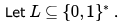Which of the following is true?

Solution:

Ans C) Every finite subset should always be regular

A set may be regular

It's all subset is not regular all the time

Say (0+1)* is regular but 0n1n is not regular

QUESTION: 4

Let L1 and L2 be languages over an alphabet £ such that L1 ⊂ L2. Which of the following is true

Solution:

Contradiction for A. Let L2 = {a,b}* ... which is regular.

And L1 = anbn which is CFL but not regular.

And here L1 is subset of L2.

Contradiction for B. Let L1 = ab ,

which is regular. And L2 = anbn which is CFL but not regular.

And here L1 is subset of L2.

C- > False , ( reason A and B).

QUESTION: 5

Language L1  is defined by the grammar: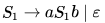Language L2  is defined by the grammar: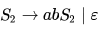Consider the following statements: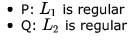Which one of the following is TRUE?

Solution: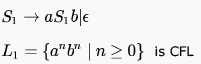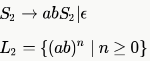is Regular having regular expression  (ab)*.

*Multiple options can be correct
QUESTION: 6

Choose the correct alternatives (More than one may be correct).

Let R1 and R2 be regular sets defined over the alphabet ∑  Then:

Solution:

Regular Languages are closed under 1. Intersection 2. Union 3. Complement 4. Kleen-Closure ∑∗−R1 is the complement of R1

B,C are true

*Multiple options can be correct
QUESTION: 7

Choose the correct alternatives (more than one may be correct) and write the corresponding letters only: Which of the following is the strongest correct statement about a finite language over some finite alphabet  ?

Solution:

(b), (c) and (d) are true. But the strongest answer would be (d) a regular language. It is trivial to say that a finite set of strings (finite language) can be accepted using a finite set of states. And regular language ⊂ context-free ⊂ contextsensitive ⊂ Turing recognizable, would imply that regular language is the strongest answer.

QUESTION: 8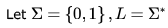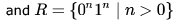then the languages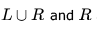are respectively

Solution: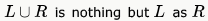is a subset of  L  and hence regular. R is deterministic context-free but not regular as we require a stack to keep the count of 0's to match that of 1's.

QUESTION: 9

Which of the following statements is false?

Solution:

Any language is a subset of ∑* which is a regular set. So, if we take any non-regular language, it is a subset of a regular language.

(a) and (c) are regular as any finite language is regular.

(d) is regular as regular set is closed under intersection.

QUESTION: 10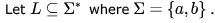Which of the following is true?

Solution:

Only D. because n and m are independent and thus no memory element required. a and b are same and are DCFL's.

c is L = { an bm | n > m }. which is not regular.
Correction:I think c should be that x has more a's than b's.

QUESTION: 11

What can be said about a regular language L over { a } whose minimal finite state automaton has two states?

Solution:

Because if we draw the minimal dfa for each of them, we will get two states each.
Whereas, {an| n>=0} requires only one state.

QUESTION: 12

Consider the following two statements: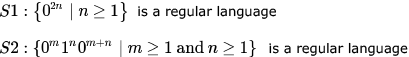Which of the following statement is correct?

Solution:

Only s1 is correct a dfa with 2 states where one of the states is both the initial and final state..

QUESTION: 13

Consider the following languages: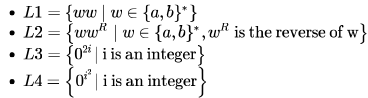Which of the languages are regular?

Solution: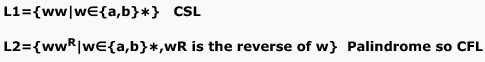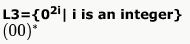Linear Power and regular expression can be stated as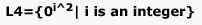non linear power So CSL

QUESTION: 14

If s is a string over (0+1)*  then let n0(s) denote the number of 0’s in s and n1(s) the number of 1’s in s. Which one of the following languages is not regular ?

Solution:

A. There are only finite 3 digit primes. And any finite set is regular

B. Here we need just 6 states to recognise L.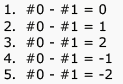If the difference goes above 2 or below -2, we go to a dead state. All other states are accepting. This transition to dead state is possible because of the words "for every prefix s' of s" in L and that is what makes this language regular.

C. L is not regular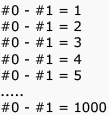All these form distinct equivalent classes under Myhill-Nerode theorem meaning from the strings in each of these sets, we can append a string which will take the new string to L, while the same string appended to string in any other set won't reach L.

For example, for 000000, we append 11, for 0000000, we append 111 etc. So, in short we need to maintain the count of 1's and 0's and the count here is not finite.

D. This is regular. We need a finite automata with 5 * 7 = 35 states for maintaining the counts of 0's mod 7 and 1's mod 5 and there cannot be more than 35 possibilities for this. With each input symbol, the transition must be going to one among these.

QUESTION: 15

Which of the following statements about regular languages is NOT true ?

Solution:

A) Every language has a regular superset: True. ∑*  is such a superset.

B) Every language has a regular subset: True. Ø is such a subset.

C) Every subset of a regular language is regular: False.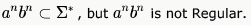D) Every subset of a finite language is regular: True. Every subset of a finite set must be finite by definition. Every finite set is regular. Hence, every subset of a finite language is regular.

QUESTION: 16

Let L be a regular language. Consider the constructions on L below: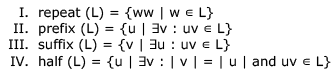Which of the constructions could lead to a non-regular language?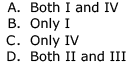Solution:

correct answer is B. only 1 .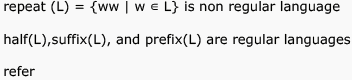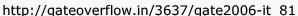QUESTION: 17

Which of the following languages is regular?

Solution:

A. CFL
B. CFL
C. Regular, language is string starting and ending with the same symbol and having length at least 3. e.g. 0x0 or 1x1
D. CFL

QUESTION: 18

Which of the following is TRUE?

Solution:

(B) Every finite subset of a non-regular set is regular.
Any finite set is always regular.

∑* being regular any non regular language is a subset of this, and hence (A) is false.

If we take a CFL which is not regular, and takes union with its complement (complement of a CFL which is not regular won't be regular as regular is closed under complement), we get ∑* which is regular. So, (C) is false.

Regular set is not closed under infinite union. Example: Let Li = {0i1i }, i ∊ N

Now, if we take infinite union over all i, we get

L = {0i1i | i ∊ N}, which is not regular. So, D is false.

QUESTION: 19

Which of the following languages is (are) non-regular?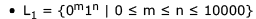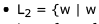reads the same forward and backward}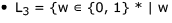contains an even number of 0's and an even number of 1's}

Solution:

L1 is regular.. since 10000 is finite.. so finite states are required..
L3 is also regular.. we can make DFA for L3.. states will represent mod 2 for 0 and mod 2 for 1, which is finite

L2 is non. regular.. it is CFG S->aSa | ... | zSz | epsilon | [a-z]

QUESTION: 20

Which of the following are regular sets?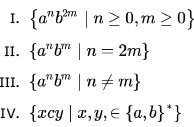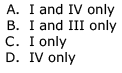Solution:

Since in option 2 and 3, n is dependent on m, therefore a comparison has to be done to evaluate those and hence are not regular.

I and IV are clearly regular sets.

QUESTION: 21

Let P be a regular language and Q be a context-free language such that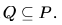(For example, let P be the language represented by the regular expression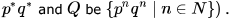Then which of the following is ALWAYS regular?

Solution:

c)complement of regular Language is regular

QUESTION: 22

Given the language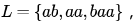which of the following strings are in L*?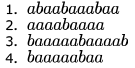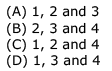Solution:

L = {ab,aa,baa}

1. abaabaaabaa    =   ab    aa   baa  ab  aa       belong to L* (combinations of strings in L)

2. aaaabaaaa        =   aa    aa  baa  aa              belong to L*

3. baaaaabaaaab  = baa  aa  ab  aa aa  b does not belong to L*

4. baaaaabaa        = baa  aa  ab  aa                 belong to L*

QUESTION: 23

Consider the languages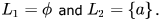Which one of the following represents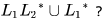Solution:

Concatenation of empty language with any language will give the empty language and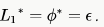Therefore,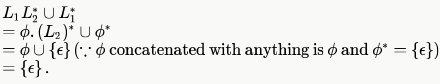Hence option (a) is True.

PS: φ   = ∈ , where ∈ is the regular expression and the language it generates is {∈}.

QUESTION: 24

Which one of the following is TRUE?

Solution:

(A) is CFL and (B) and (D) are CSL. (C) is regular and regular expression for (C) would be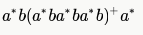QUESTION: 25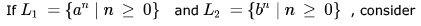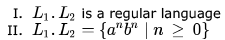Which one of the following is CORRECT?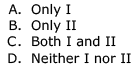Solution: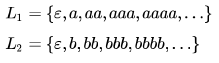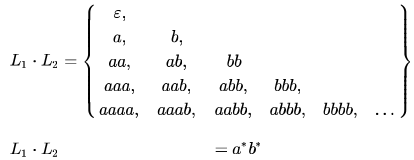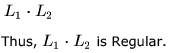(Also, since both L1 and L2 are Regular, their concatenation has to be Regular since Regular languages are closed under concatenation)

However, L1.L2 ≠anbn . This is because in a*b* , the number of 's and 's can be different whereas in  they have to be the same.

QUESTION: 26

Which of the following is/are regular languages?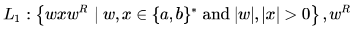,  is the reverse of string ω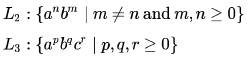Solution:

L3 is simple to guess, it is regular. Below is DFA for L1.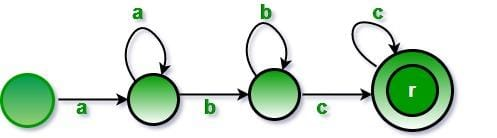L1 is interesting. The important thing to note about L1 is length of x is greater than 0, i.e., |x| > 0. So any string than starts and ends with same character is acceptable by language and remaining string becomes w. Below is DFA for L1.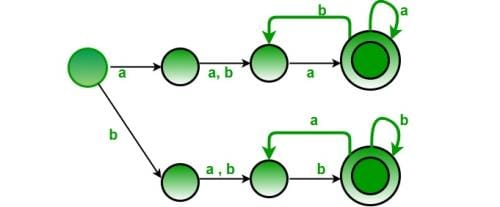QUESTION: 27

Consider the following three statements:

(i) Intersection of infinitely many regular languages must be regular.

(ii) Every subset of a regular language is regular.

(iii) If L is regular and M is not regular then L.M is necessarily not regular.

Which of the following gives the correct true/false evaluation of the above?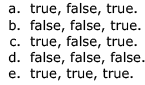Solution:

i) False

Regular Languages are not closed under Infinite Union and Intersection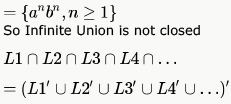As Infinite Union is not closed , So Infinite Intersection is also not closed

ii) False.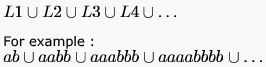iii) False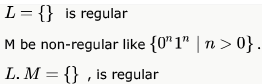QUESTION: 28

Let B  consist of all binary strings beginning with a 1 whose value when converted to decimal is divisible by 7 .

Solution: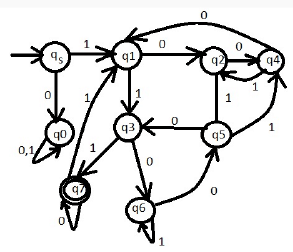QUESTION: 29

Which one of the following languages over the alphabet 0,1 is regular?

Solution:

L = { 0^ m2  : m>= 3}

Now, in L* if we can generate 9 continuous powers of zero, then further every power can be generated by concatenating  09.

Here , L = {09 ,016, 025 , ...}

So, here are 9 continuous powers:

0120 : 016 016 016 09 09 09 09 09 09 09 09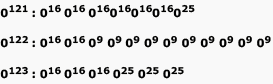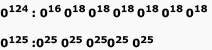0126 : 018018018018018018018    {018 can be generated as 09 09 }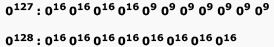Now, 0129  can be given as 0120 09 and so on..
Every Further powers can be generated by concatenating  09.

QUESTION: 30

Identify the regular expression which represents the language containing all strings of a's and b's where each string contains at least two b's

Solution:Use Code STAYHOME200 and get INR 200 additional OFF Use Coupon Code